# Operators in C#

## Operators in C# with Examples

In this article, I am going to discuss Operators in C# with Examples. Please read our previous article, where we discussed Variables in C# with Examples. The Operators are the foundation of any programming language. Thus, the functionality of the C# language is incomplete without the use of operators. At the end of this article, you will understand what are Operators and when, and how to use them in C# Application with examples.

##### What are Operators in C#?

Operators in C# are symbols that are used to perform operations on operands. For example, consider the expression 2 + 3 = 5, here 2 and 3 are operands, and + and = are called operators. So, the Operators in C# are used to manipulate the variables and values in a program.

int x = 10, y = 20;
int result1 = x + y; //Operator Manipulating Variables, where x and y are variables and + is operator
int result2 = 10 + 20; //Operator Manipulating Values, where 10 and 20 are value and + is operator

Note: In the above example, x, y, 10, and 20 are called Operands. So, the operand may be variables or values.

##### Types of Operators in C#:

The Operators are classified based on the type of operations they perform on operands in C# language. They are as follows:

1. Arithmetic Operators
2. Relational Operators
3. Logical Operators
4. Bitwise Operators
5. Assignment Operators
6. Unary Operators or
7. Ternary Operator or Conditional Operator

In C#, the Operators can also be categorized based on the Number of Operands:

1. Unary Operator: The Operator that requires one operand (variable or value) to perform the operation is called Unary Operator.
2. Binary Operator: Then Operator that requires two operands (variables or values) to perform the operation is called Binary Operator.
3. Ternary Operator: The Operator that requires three operands (variables or values) to perform the operation is called Ternary Operator. The Ternary Operator is also called Conditional Operator.

For a better understanding of the different types of operators supported in C# Programming Language, please have a look at the below image.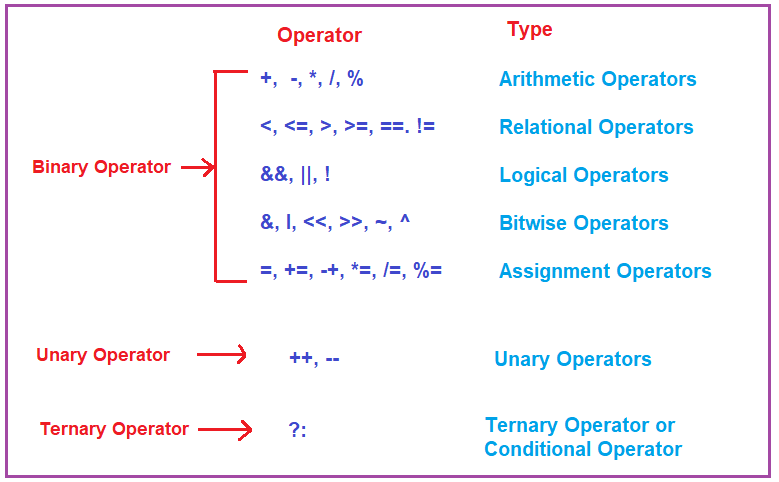##### Arithmetic Operators in C#

The Arithmetic Operators in C# are used to perform arithmetic/mathematical operations like addition, subtraction, multiplication, division, etc. on operands. The following Operators are falling into this category.

The + operator adds two operands. As this operator works with two operands, so, this + (plus) operator belongs to the category of the binary operator. The + Operator adds the left-hand side operand value with the right-hand side operand value and returns the result. For example:
int a=10;
int b=5;
int c = a+b; //15, Here, it will add the a and b operand values i.e. 10 + 5

Subtraction Operator (-):
The – operator subtracts two operands. As this operator works with two operands, so, this – (minus) operator belongs to the category of the binary operator. The Minus Operator substracts the left-hand side operand value from the right-hand side operand value and returns the result. For example:
int a=10;
int b=5;
int c = a-b; //5, Here, it will subtract b from a i.e. 10 – 5

Multiplication Operator (*):
The * (Multiply) operator multiplies two operands. As this operator works with two operands, so, this * (Multiply) operator belongs to the category of the binary operator. The Multiply Operator multiplies the left-hand side operand value with the right-hand side operand value and returns the result. For example:
int a=10;
int b=5;
int c=a*b; //50, Here, it will multiply a with b i.e. 10 * 5

Division Operator (/):
The / (Division) operator divides two operands. As this operator works with two operands, so, this / (Division) operator belongs to the category of the binary operator. The Division Operator divides the left-hand side operand value with the right-hand side operand value and returns the result. For example:
int a=10;
int b=5;
int c=a/b; //2, Here, it will divide 10 / 5

Modulus Operator (%):
The % (Modulos) operator returns the remainder when the first operand is divided by the second. As this operator works with two operands, so, this % (Modulos) operator belongs to the category of the binary operator. For example:
int a=10;
int b=5;
int c=a%b; //0, Here, it will divide 10 / 5 and it will return the remainder which is 0 in this case

##### Example to Understand Arithmetic Operators in C#:

In the below example, I am showing how to use Arithmetic Operators with Operand which are variables. Here, Num1 and Num2 are variables and all the Arithmetic Operators are working on these two variables.

```using System;
namespace OperatorsDemo
{
class Program
{
static void Main(string[] args)
{
int Result;
int Num1 = 20, Num2 = 10;

Result = (Num1 + Num2);

// Subtraction Operation
Result = (Num1 - Num2);
Console.WriteLine(\$"Subtraction Operator: {Result}");

// Multiplication Operation
Result = (Num1 * Num2);
Console.WriteLine(\$"Multiplication Operator: {Result}");

// Division Operation
Result = (Num1 / Num2);
Console.WriteLine(\$"Division Operator: {Result}");

// Modulo Operation
Result = (Num1 % Num2);
Console.WriteLine(\$"Modulo Operator: {Result}");

}
}
}
```
###### Output: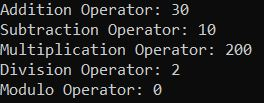In the following example, I am showing how to use Arithmetic Operators with Operand which are values. Here, 10 and 20 are values and all the Arithmetic Operators are working on these two values.

```using System;
namespace OperatorsDemo
{
class Program
{
static void Main(string[] args)
{
int Result;
// int Num1 = 20, Num2 = 10;

Result = 20 + 10;

// Subtraction Operation
Result = 20 - 10;
Console.WriteLine(\$"Subtraction Operator: {Result}");

// Multiplication Operation
Result = 20 * 10;
Console.WriteLine(\$"Multiplication Operator: {Result}");

// Division Operation
Result = 20 / 10;
Console.WriteLine(\$"Division Operator: {Result}");

// Modulo Operation
Result = 20 % 10;
Console.WriteLine(\$"Modulo Operator: {Result}");

}
}
}
```
###### Output:Note: The point that you need to remember is that the operator working on the operands and the operand may be variables, or values and can also be the combination of both.

##### Assignment Operators in C#:

The Assignment Operators in C# are used to assign a value to a variable. The left-hand side operand of the assignment operator is a variable and the right-hand side operand of the assignment operator can be a value or an expression that must return some value and that value is going to assign to the left-hand side variable.

The most important point that you need to keep in mind is that the value on the right-hand side must be of the same data type as the variable on the left-hand side else you will get a compile-time error. The different Types of Assignment Operators supported in the C# language are as follows:

###### Simple Assignment (=):

This operator is used to assign the value of the right-hand side operand to the left-hand side operand i.e. to a variable.
For example:
int a=10;
int b=20;
char ch = ‘a’;
a=a+4; //(a=10+4)
b=b-4; //(b=20-4)

This operator is the combination of + and = operators. It is used to add the left-hand side operand value with the right-hand side operand value and then assign the result to the left-hand side variable.
For example:
int a=5;
int b=6;
a += b; //a=a+b; That means (a += b) can be written as (a = a + b)

###### Subtract Assignment (-=):

This operator is the combination of – and = operators. It is used to subtract the right-hand side operand value from the left-hand side operand value and then assign the result to the left-hand side variable.
For example:
int a=10;
int b=5;
a -= b; //a=a-b; That means (a -= b) can be written as (a = a – b)

###### Multiply Assignment (*=):

This operator is the combination of * and = operators. It is used to multiply the left-hand side operand value with the right-hand side operand value and then assign the result to the left-hand side variable.
For example:
int a=10;
int b=5;
a *= b; //a=a*b; That means (a *= b) can be written as (a = a * b)

###### Division Assignment (/=):

This operator is the combination of / and = operators. It is used to divide the left-hand side operand value with the right-hand side operand value and then assign the result to the left-hand side variable.
For example:
int a=10;
int b=5;
a /= b; //a=a/b; That means (a /= b) can be written as (a = a / b)

###### Modulus Assignment (%=):

This operator is the combination of % and = operators. It is used to divide the left-hand side operand value with the right-hand side operand value and then assigns the remainder of this division to the left-hand side variable.
For example:
int a=10;
int b=5;
a %= b; //a=a%b; That means (a %= b) can be written as (a = a % b)

##### Example to Understand Assignment Operators in C#:
```using System;
namespace OperatorsDemo
{
class Program
{
static void Main(string[] args)
{
// Initialize variable x using Simple Assignment Operator "="
int x = 15;

x += 10;  //It means x = x + 10 i.e. 15 + 10 = 25

// initialize variable x again
x = 20;
x -= 5;  //It means x = x - 5 i.e. 20 - 5 = 15
Console.WriteLine(\$"Subtract Assignment Operator: {x}");

// initialize variable x again
x = 15;
x *= 5; //It means x = x * 5  i.e. 15 * 5 = 75
Console.WriteLine(\$"Multiply Assignment Operator: {x}");

// initialize variable x again
x = 25;
x /= 5; //It means x = x / 5 i.e. 25 / 5 = 5
Console.WriteLine(\$"Division Assignment Operator: {x}");

// initialize variable x again
x = 25;
x %= 5; //It means x = x % 5 i.e. 25 % 5 = 0
Console.WriteLine(\$"Modulo Assignment Operator: {x}");

}
}
}
```
###### Output: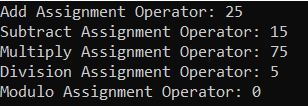##### Relational Operators in C#:

The Relational Operators in C# are also known as Comparison Operators. It determines the relationship between two operands and returns the Boolean results, i.e. true or false after the comparison. The Different Types of Relational Operators supported by C# are as follows.

###### Equal to (==):

This Operator is used to return true if the left-hand side operand value is equal to the right-hand side operand value. For example, 5==3 is evaluated to be false. So, this Equal to (==) operator will check whether the two given operand values are equal or not. If equal returns true else returns false.

###### Not Equal to (!=):

This Operator is used to return true if the left-hand side operand value is not equal to the right-hand side operand value. For example, 5!=3 is evaluated to be true. So, this Not Equal to (!=) operator will check whether the two given operand values are equal or not. If equal returns false else returns true.

###### Less than (<):

This Operator is used to return true if the left-hand side operand value is less than the right-hand side operand value. For example, 5<3 is evaluated to be false. So, this Less than (<) operator will check whether the first operand value is less than the second operand value or not. If so, returns true else returns false.

###### Less than or equal to (<=):

This Operator is used to return true if the left-hand side operand value is less than or equal to the right-hand side operand value. For example, 5<=5 is evaluated to be true. So. this Less than or equal to (<=) operator will check whether the first operand value is less than or equal to the second operand value. If so returns true else returns false.

###### Greater than (>):

This Operator is used to return true if the left-hand side operand value is greater than the right-hand side operand value. For example, 5>3 is evaluated to be true. So, this Greater than (>) operator will check whether the first operand value is greater than the second operand value. If so, returns true else return false.

###### Greater than or Equal to (>=):

This Operator is used to return true if the left-hand side operand value is greater than or equal to the right-hand side operand value. For example, 5>=5 is evaluated to be true. So, this Greater than or Equal to (>=) operator will check whether the first operand value is greater than or equal to the second operand value. If so, returns true else returns false.

##### Example to Understand Relational Operators in C#:
```using System;
namespace OperatorsDemo
{
class Program
{
static void Main(string[] args)
{
bool Result;
int Num1 = 5, Num2 = 10;

// Equal to Operator
Result = (Num1 == Num2);
Console.WriteLine("Equal (=) to Operator: " + Result);

// Greater than Operator
Result = (Num1 > Num2);
Console.WriteLine("Greater (<) than Operator: " + Result);

// Less than Operator
Result = (Num1 < Num2);
Console.WriteLine("Less than (>) Operator: " + Result);

// Greater than Equal to Operator
Result = (Num1 >= Num2);
Console.WriteLine("Greater than or Equal to (>=) Operator: " + Result);

// Less than Equal to Operator
Result = (Num1 <= Num2);
Console.WriteLine("Lesser than or Equal to (<=) Operator: " + Result);

// Not Equal To Operator
Result = (Num1 != Num2);
Console.WriteLine("Not Equal to (!=) Operator: " + Result);

}
}
}
```
###### Output: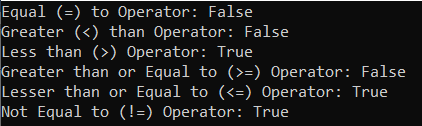##### Logical Operators in C#:

The Logical Operators are mainly used in conditional statements and loops for evaluating a condition. These operators are going to work with boolean expressions. The different types of Logical Operators supported in C# are as follows:

##### Logical OR (||):

This operator is used to return true if either of the Boolean expressions is true. For example, false || true is evaluated to be true. That means the Logical OR (||) operator returns true when one (or both) of the conditions in the expression is satisfied. Otherwise, it will return false. For example, a || b returns true if either a or b is true. Also, it returns true when both a and b are true.

##### Logical AND (&&):

This operator is used to return true if all the Boolean Expressions are true. For example, false && true is evaluated to be false. That means the Logical AND (&&) operator returns true when both the conditions in the expression are satisfied. Otherwise, it will return false. For example, a && b return true only when both a and b are true.

##### Logical NOT (!):

This operator is used to return true if the condition in the expression is not satisfied. Otherwise, it will return false. For example, !a returns true if a is false.

##### Example to Understand Logical Operators in C#:
```using System;
namespace OperatorsDemo
{
class Program
{
static void Main(string[] args)
{
bool x = true, y = false, z;

//Logical AND operator
z = x && y;
Console.WriteLine("Logical AND Operator (&&) : " + z);

//Logical OR operator
z = x || y;
Console.WriteLine("Logical OR Operator (||) : " + z);

//Logical NOT operator
z = !x;
Console.WriteLine("Logical NOT Operator (!) : " + z);

}
}
}
```
###### Output: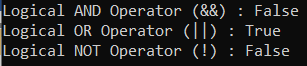##### Bitwise Operators in C#:

The Bitwise Operators in C# perform bit-by-bit processing. They can be used with any of the integer (short, int, long, ushort, uint, ulong, byte) types. The different types of Bitwise Operators supported in C# are as follows.

###### Bitwise OR (|)

Bitwise OR operator is represented by |. This operator performs the bitwise OR operation on the corresponding bits of the two operands involved in the operation. If either of the bits is 1, it gives 1. If not, it gives 0.
For example,
int a=12, b=25;
int result = a|b; //29
How?
12 Binary Number: 00001100
25 Binary Number: 00011001
Bitwise OR operation between 12 and 25:
00001100
00011001
========
00011101 (it is 29 in decimal)
Note: If the operands are of type bool, the bitwise OR operation is equivalent to the logical OR operation between them.

###### Bitwise AND (&):

Bitwise OR operator is represented by &. This operator performs the bitwise AND operation on the corresponding bits of two operands involved in the operation. If both of the bits are 1, it gives 1. If either of the bits is not 1, it gives 0.
For example,
int a=12, b=25;
int result = a&b; //8
How?
12 Binary Number: 00001100
25 Binary Number: 00011001
Bitwise AND operation between 12 and 25:
00001100
00011001
========
00001000 (it is 8 in decimal)
Note: If the operands are of type bool, the bitwise AND operation is equivalent to the logical AND operation between them.

###### Bitwise XOR (^):

The bitwise OR operator is represented by ^. This operator performs a bitwise XOR operation on the corresponding bits of two operands. If the corresponding bits are different, it gives 1. If the corresponding bits are the same, it gives 0.
For example,
int a=12, b=25;
int result = a^b; //21
How?
12 Binary Number: 00001100
25 Binary Number: 00011001
Bitwise AND operation between 12 and 25:
00001100
00011001
========
00010101 (it is 21 in decimal)

##### Example to Understand Bitwise Operators in C#:
```using System;
namespace OperatorsDemo
{
class Program
{
static void Main(string[] args)
{
int a = 12, b = 25, Result;

// Bitwise AND Operator
Result = a & b;
Console.WriteLine(\$"Bitwise AND: {Result}");

// Bitwise OR Operator
Result = a | b;
Console.WriteLine(\$"Bitwise OR: {Result}");

// Bitwise XOR Operator
Result = a ^ b;
Console.WriteLine(\$"Bitwise XOR: {Result}");

}
}
}
```
###### Output: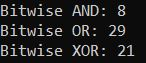In the above example, we are using BIT Wise Operators with integer data type and hence it performs the Bitwise Operations. But, if use BIT-wise Operators with boolean data types, then these bitwise operators AND, OR, and XOR behaves like Logical AND, and OR operations. For a better understanding, please have a look at the below example. In the below example, we are using the BIT-wise operators on boolean operands and hence they are going to perform the Logical AND, OR, and XOR Operations.

```using System;
namespace OperatorsDemo
{
class Program
{
static void Main(string[] args)
{
bool a = true, b = false, Result;

// Bitwise AND Operator
Result = a & b;
Console.WriteLine(\$"Bitwise AND: {Result}");

// Bitwise OR Operator
Result = a | b;
Console.WriteLine(\$"Bitwise OR: {Result}");

// Bitwise XOR Operator
Result = a ^ b;
Console.WriteLine(\$"Bitwise XOR: {Result}");

}
}
}
```
###### Output: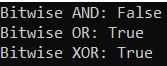Note: The point that you need to remember while working with BIT-Wise Operator is that, depending on the operand on which they are working, the behavior is going to change. It means if they are working with integer operands, they will work like bitwise operators and return the result as an integer and if they are working with boolean operands, then work like logical operators and return the result as a boolean.

##### Unary Operators in C#:

The Unary Operators in C# need only one operand. They are used to increment or decrement a value. There are two types of Unary Operators. They are as follows:

1. Increment operators (++): Example: (++x, x++)
2. Decrement operators (–): Example: (–x, x–)
##### Increment Operator (++) in C# Language:

The Increment Operator (++) is a unary operator. It operates on a single operand only. Again, it is classified into two types:

1. Post-Increment Operator
2. Pre-Increment Operator
##### Post Increment Operators:

The Post Increment Operators are the operators that are used as a suffix to its variable. It is placed after the variable. For example, a++ will also increase the value of the variable a by 1.

Syntax: Variable++;
Example: x++;

##### Pre-Increment Operators:

The Pre-Increment Operators are the operators which are used as a prefix to its variable. It is placed before the variable. For example, ++a will increase the value of the variable a by 1.

Syntax: ++Variable;
Example: ++x;

##### Decrement Operators in C# Language:

The Decrement Operator (–) is a unary operator. It takes one value at a time. It is again classified into two types. They are as follows:

1. Post Decrement Operator
2. Pre-Decrement Operator
##### Post Decrement Operators:

The Post Decrement Operators are the operators that are used as a suffix to its variable. It is placed after the variable. For example, a– will also decrease the value of the variable a by 1.

Syntax: Variable–;
Example: x–;

##### Pre-Decrement Operators:

The Pre-Decrement Operators are the operators that are a prefix to its variable. It is placed before the variable. For example, –a will decrease the value of the variable a by 1.

Syntax: –Variable;
Example: x;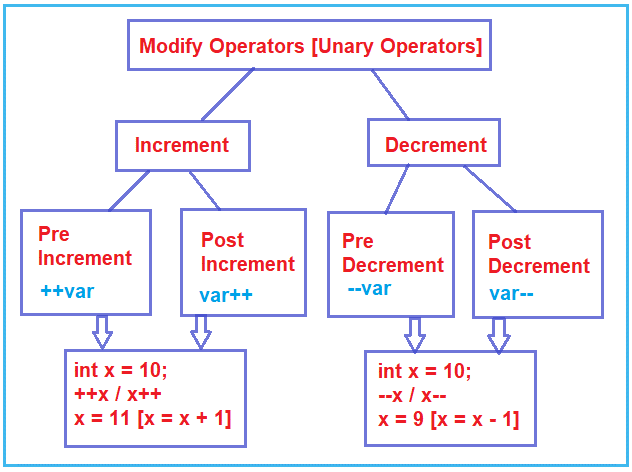Note: Increment Operator means to increment the value of the variable by 1 and Decrement Operator means to decrement the value of the variable by 1.

##### Example to Understand Increment Operators in C# Language:
```using System;
namespace OperatorsDemo
{
class Program
{
static void Main(string[] args)
{
// Post-Increment
int x = 10;
// Result1 is assigned 10 only,
// x is not updated yet
int Result1 = x++;
//x becomes 11 now
Console.WriteLine("x is {0} and Result1 is {1}", x, Result1);

// Pre-Increment
int y = 10;
int Result2 = ++y;
//y and Result2 have same values = 11
Console.WriteLine("y is {0} and Result2 is {1}", y, Result2);

}
}
}
```
###### Output: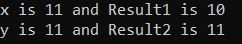##### Example to understand Decrement Operators in C# Language:
```using System;
namespace OperatorsDemo
{
class Program
{
static void Main(string[] args)
{
// Post-Decrement
int x = 10;
// Result1 is assigned 10 only,
// x is not yet updated
int Result1 = x--;
//x becomes 9 now
Console.WriteLine("x is {0} and Result1 is {1}", x, Result1);

// Pre-Decrement
int y = 10;
int Result2 = --y;
//y and Result2 have same values i.e. 9
Console.WriteLine("y is {0} and Result2 is {1}", y, Result2);

}
}
}
```
###### Output: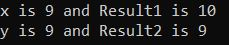##### Five Steps to Understand How the Unary Operators Works in C#?

I see, many of the students and developers getting confused when they use increment and decrement operators in an expression. To make you understand how exactly the unary ++ and — operators work in C#, we need to follow 5 simple steps. The steps are shown in the below diagram.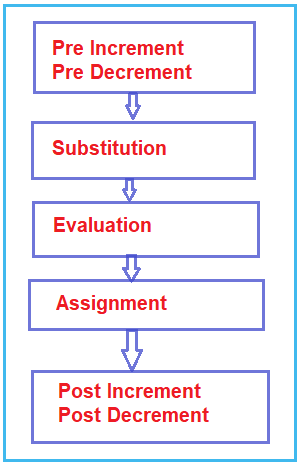1. Step 1: If there is some pre-increment or pre-decrement in the expression, that should execute first.
2. Step 2: The second step is to substitute the values in the expression.
3. Step 3: In the third step we need to evaluate the expression.
4. Step 4: In the fourth step Assignment needs to be performed.
5. Step 5: The final step is to perform post-increment or post-decrement.

Now, if you have still doubt about the above five steps, then don’t worry we will see some examples to understand this step in a better way.

##### Example to Understand Increment and Decrement Operators in C# Language:

Let us see one complex example to understand this concept. Please have a look at the following example. Here, we are declaring three variables x, y, and z, and then evaluating the expression as z = x++ * –y; finally, we are printing the value of x, y, and z in the console.

```using System;
namespace OperatorsDemo
{
class Program
{
static void Main(string[] args)
{
int x = 10, y = 20, z;
z = x++ * --y;
Console.WriteLine(\$"x={x}, y={y}, z={z}");
}
}
}
```

Let us evaluate the expression z = x++ * –y; by following the above 5 steps:

1. The First step is Pre-Increment or Pre-Decrement. Is there any pre-increment or pre-decrement in the expression? There is no pre-increment but there is a pre-decrement in the expression i.e. –y. So, execute that pre-decrement operator which will decrease the value of y by 1 i.e. now y becomes 19.
2. The second step is Substitution. So, substitute the values of x and y. That means x will be substituted by 10 and y will be substituted by 19.
3. The third step is Evaluation. So, evaluate the expression i.e. 10 * 19 = 190.
4. The fourth step is the Assignment. So, assign the evaluated value to the given variable i.e. 190 will be assigned to z. So, now the z value becomes 190.
5. The last step is Post-Increment and Post-Decrement. Is there any post-increment or post-decrement in the expression? There is no post-decrement but there is a post-increment in the expression i.e. x++. So, execute that post-increment which will increase the value of x by 1 i.e. x becomes 11.

So, when you will execute the above program it will print the x, y, and z values as 11, 19, and 190 respectively.

Note: It is not recommended by Microsoft to use the ++ or — operators inside a complex expression like the above example. The reason is if we use the ++ or — operator on the same variable multiple times in an expression, then we cannot predict the output. So, if you are just incrementing the value of a variable by 1 or decrementing the variable by 1, then in that scenario you need to use these Increment or Decrement Operators. One of the ideal scenarios where you need to use the increment or decrement operator is inside a loop. What is a loop, why loop, and what is a counter variable, we will discuss this in our upcoming articles, but now just have a look at the following example, where I am using the for loop and increment operator?

```using System;
namespace OperatorsDemo
{
class Program
{
static void Main(string[] args)
{
for (int i = 0; i < 10; i++)
{
Console.WriteLine(i);
}
}
}
}```
##### Ternary Operator in C#:

The Ternary Operator in C# is also known as the Conditional Operator (?:). It is actually the shorthand of the if-else statement. It is called ternary because it has three operands or arguments. The first argument is a comparison argument, the second is the result of a true comparison, and the third is the result of a false comparison.

Syntax: Condition? first_expression : second_expression;

The above statement means that first, we need to evaluate the condition. If the condition is true the first_expression is executed and becomes the result and if the condition is false, the second_expression is executed and becomes the result.

##### Example to understand Ternary Operator in C#:
```using System;
namespace OperatorsDemo
{
class Program
{
static void Main(string[] args)
{
int a = 20, b = 10, res;
res = ((a > b) ?a : b);
Console.WriteLine("Result = " + res);

}
}
}
```

Output: Result = 20

1.